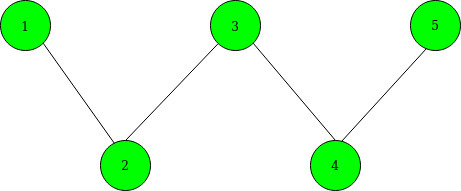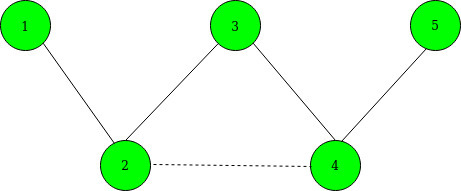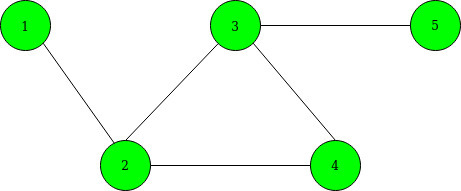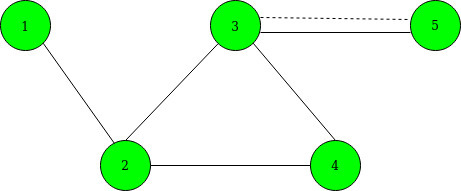# Maximize shortest path between given vertices by adding a single edge

Given an undirected graph of N nodes and M vertices. You are also given a K edges as selected[]. The task to maximize the shortest path length between node 1 to node N by adding single edges between any two vertices from the given selected edges.
Note: You may add an edge between any two selected vertices who have already an edge between them.

Input: N = 5, M = 4, K = 2, selected[] = {2, 4}
Below is the given graph:Output: 3
Explanation:
Before adding an edge between 2 and 4, the Shortest Path becomes: 1–>2–>3–>4–>5.
After adding an edge between 2 and 4, the Shortest Path becomes 1–>2–>4–>5. Below is the graph after adding edges. denoted by the dashed line.Input: N = 5 M = 5 K = 3 selected[] = {1, 3, 5}
Below is the given graph:Output: 3
Explanation:
We can add an edge between 3 and 5 as they have already an edge between them. so, the shortest path becomes 1–>2–>3–>5. Below is the graph after adding edges. denoted by the dashed line.## Recommended: Please try your approach on {IDE} first, before moving on to the solution.

Approach: The idea is to use Breadth-First Search to find the distance from vertices 1 and N to each selected vertex. For selected vertex i, let xi denote the distance to node 1, and yi denote the distance to node N. Below are the steps:

1. Maintain a 2D matrix(say dist[]) having 2 rows and N columns.
2. In the first row, Maintain the shortest distance between node 1 and other vertices in the graph using BFS Traversal.
3. In the second row, Maintain the shortest distance between node N and the other vertices of the graph using BFS Traversal.
4. Now, choose two selected vertices a and b from selected[] to minimize the value of min(xa + yb, ya + xb). For this do the following:
• Create a vector of pairs and store the value of (xi – yi) with their respective selected node.
• Sort the above vector of pairs.
• Initialize best to 0 and max to -INF.
• Now traverse the above vector of pairs and for each selected node(say a) update the value of best to maximum of (best, max + dist[a]) and update max to maximum of (max, dist[a]).
5. After the above operations the maximum of (dist[N-1] and best + 1) given the minimum shortest path.

Below is the implementation of the above approach:

## CPP

 `// C++ program for the above approach ` `#include ` `using` `namespace` `std; ` `const` `int` `INF = 1e9 + 7; ` `int` `N, M; ` ` `  `// To store graph as adjacency list ` `vector<``int``> edges; ` ` `  `// To store the shortest path ` `int` `dist; ` ` `  `// Function that performs BFS Traversal ` `void` `bfs(``int``* dist, ``int` `s) ` `{ ` `    ``int` `q; ` ` `  `    ``// Fill initially each distance as INF ` `    ``fill(dist, dist + N, INF); ` `    ``int` `qh = 0, qt = 0; ` `    ``q[qh++] = s; ` `    ``dist[s] = 0; ` ` `  `    ``// Perform BFS ` `    ``while` `(qt < qh) { ` ` `  `        ``int` `x = q[qt++]; ` ` `  `        ``// Traverse the current edges ` `        ``for` `(``int` `y : edges[x]) { ` `            ``if` `(dist[y] == INF) { ` ` `  `                ``// Update the distance ` `                ``dist[y] = dist[x] + 1; ` ` `  `                ``// Insert in queue ` `                ``q[qh++] = y; ` `            ``} ` `        ``} ` `    ``} ` `} ` ` `  `// Function that maximizes the shortest ` `// path between source and destination ` `// vertex by adding a single edge between ` `// given selected nodes ` `void` `shortestPathCost(``int` `selected[], ``int` `K) ` `{ ` `    ``vector > data; ` ` `  `    ``// To update the shortest distance ` `    ``// between node 1 to other vertices ` `    ``bfs(dist, 0); ` ` `  `    ``// To update the shortest distance ` `    ``// between node N to other vertices ` `    ``bfs(dist, N - 1); ` ` `  `    ``for` `(``int` `i = 0; i < K; i++) { ` ` `  `        ``// Store the values x[i] - y[i] ` `        ``data.emplace_back(dist[selected[i]] ` `                              ``- dist[selected[i]], ` `                          ``selected[i]); ` `    ``} ` ` `  `    ``// Sort all the vectors of pairs ` `    ``sort(data.begin(), data.end()); ` `    ``int` `best = 0; ` `    ``int` `MAX = -INF; ` ` `  `    ``// Traverse data[] ` `    ``for` `(``auto` `it : data) { ` `        ``int` `a = it.second; ` `        ``best = max(best, ` `                   ``MAX + dist[a]); ` ` `  `        ``// Maximize x[a] - y[b] ` `        ``MAX= max(MAX, dist[a]); ` `    ``} ` ` `  `    ``// Print minimum cost ` `    ``printf``(``"%d\n"``, min(dist[N - 1], best + 1)); ` `} ` ` `  `// Driver Code ` `int` `main() ` `{ ` `    ``// Given nodes and edges ` `    ``N = 5, M = 4; ` `    ``int` `K = 2; ` `    ``int` `selected[] = { 1, 3 }; ` ` `  `    ``// Sort the selected nodes ` `    ``sort(selected, selected + K); ` ` `  `    ``// Given edges ` `    ``edges.push_back(1); ` `    ``edges.push_back(0); ` `    ``edges.push_back(2); ` `    ``edges.push_back(1); ` `    ``edges.push_back(3); ` `    ``edges.push_back(2); ` `    ``edges.push_back(4); ` `    ``edges.push_back(3); ` ` `  `    ``// Function Call ` `    ``shortestPathCost(selected, K); ` `    ``return` `0; ` `} `

Output:

```3
```

Time Complexity: O(N*log N + M)
Auxiliary Space: O(N)

Attention reader! Don’t stop learning now. Get hold of all the important DSA concepts with the DSA Self Paced Course at a student-friendly price and become industry ready.

My Personal Notes arrow_drop_upCheck out this Author's contributed articles.

If you like GeeksforGeeks and would like to contribute, you can also write an article using contribute.geeksforgeeks.org or mail your article to contribute@geeksforgeeks.org. See your article appearing on the GeeksforGeeks main page and help other Geeks.

Please Improve this article if you find anything incorrect by clicking on the "Improve Article" button below.